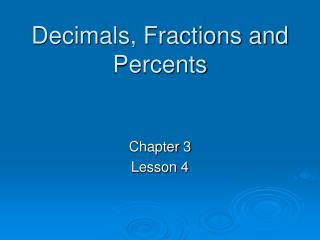DownloadDownload PresentationDecimals, Fractions and Percents

# Decimals, Fractions and Percents

Télécharger la présentation## Decimals, Fractions and Percents

- - - - - - - - - - - - - - - - - - - - - - - - - - - E N D - - - - - - - - - - - - - - - - - - - - - - - - - - -
##### Presentation Transcript

1. Decimals, Fractions and Percents Chapter 3 Lesson 4

2. % % fraction Put # over 100 Reduce the fraction Decimal Fraction

3. % fraction Example: 16% You try: 64% 81%

4. % fraction % Add % Solve by cross products Set fraction = to Decimal Fraction

5. fraction % Example: You try:

6. % % decimal Move decimal 2 places left Decimal Fraction

7. % decimal Example: 34% 34 .34 You try: 68% =.68 3.6% =.036

8. % decimal % Move decimal 2 places right Decimal Fraction

9. decimal % Example: .84 84 84% You try: .92 =92% 3.65 =365% .0297 =2.97%

10. % decimal fraction Put # over its last digits place value Reduce Decimal Fraction

11. decimal fraction Example: .235 tenths hundredths thousandths You try: .72 6.7

12. % fraction decimal Decimal Fraction Divide numerator by denominator

13. fraction decimal Example: You try: 1 2 5 =.833 - 8 20 - 16 =.8 40 -40 = .125

14. % Put # over 100 Add % Solve by cross products Move decimal 2 places right Move decimal 2 places left Reduce the fraction Set fraction = to Put # over its last digits place value Reduce Decimal Fraction Divide numerator by denominator